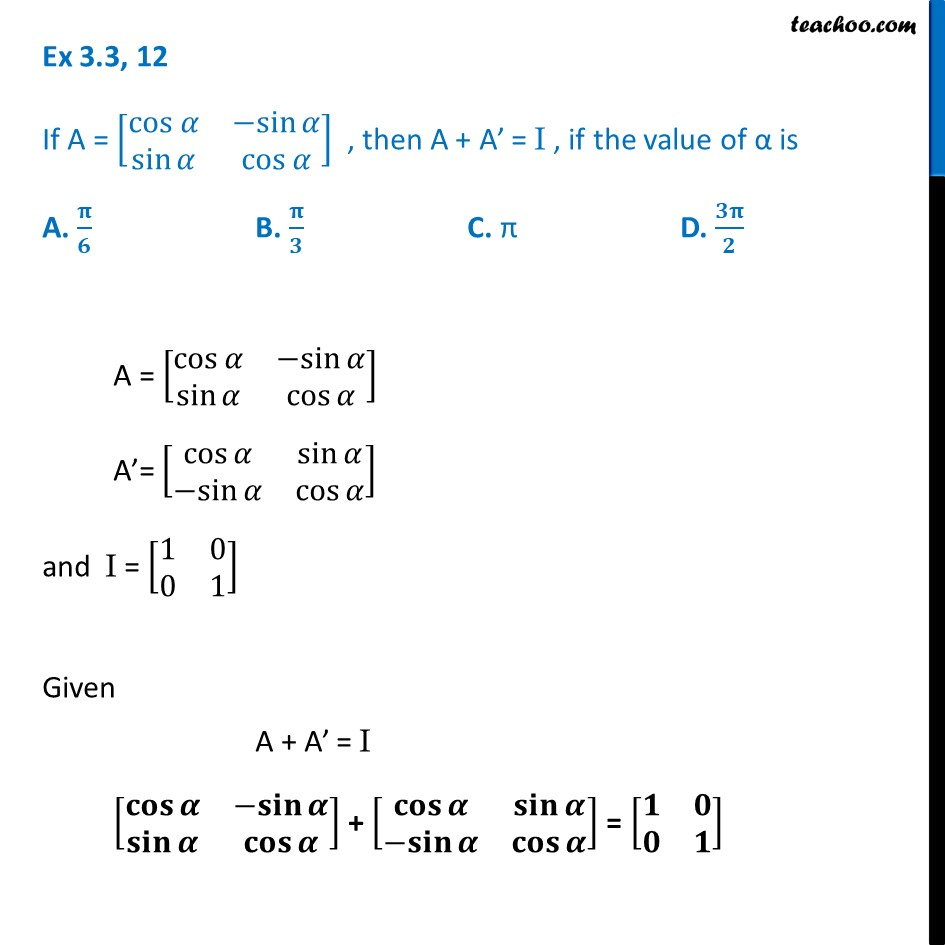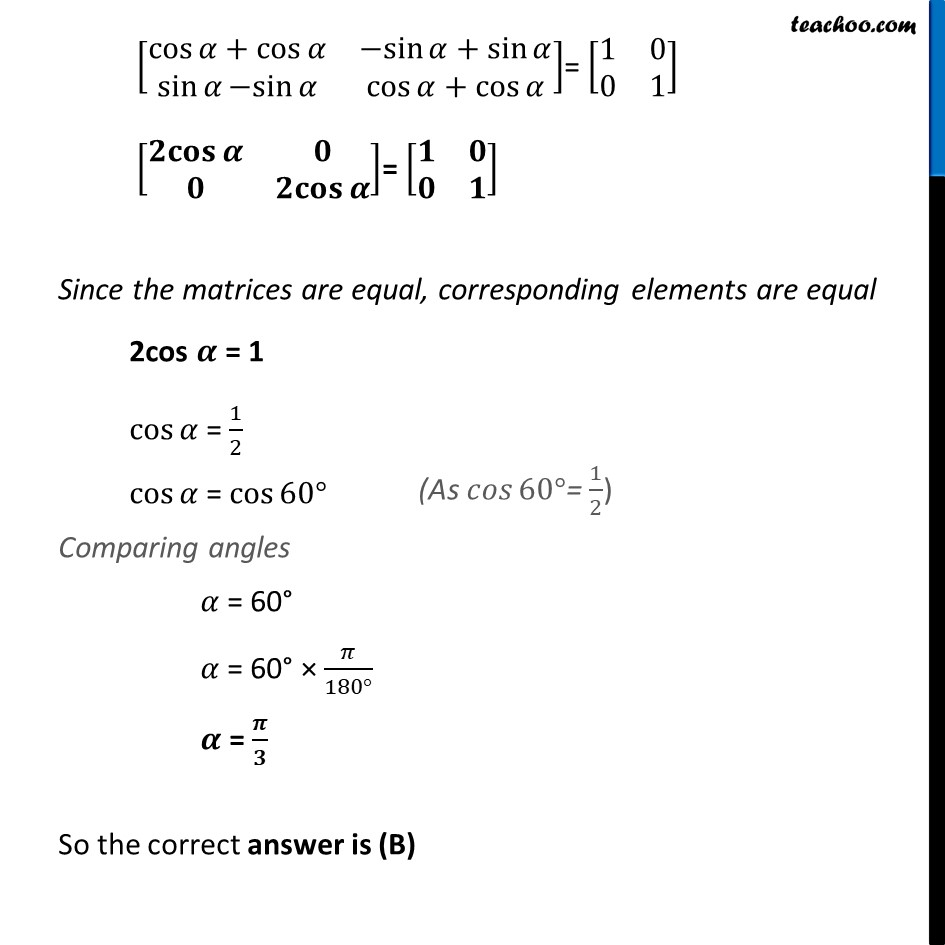Ex 3.3

Chapter 3 Class 12 Matrices
Serial order wiseLearn in your speed, with individual attention - Teachoo Maths 1-on-1 Class

### Transcript

Ex 3.3, 12 If A = [■8(cos 𝛼&〖−sin〗⁡𝛼@sin⁡𝛼&cos⁡𝛼 )] , then A + A’ = I , if the value of α is A. 𝛑/𝟔 B. 𝛑/𝟑 C. π D. 𝟑𝛑/𝟐 A = [■8(cos⁡𝛼&〖−sin〗⁡𝛼@sin⁡𝛼&cos⁡𝛼 )] A’= [■8(cos⁡𝛼&sin⁡𝛼@〖−sin〗⁡𝛼&cos⁡𝛼 )] and I = [■8(1&0@0&1)] Given A + A’ = I [■8(𝐜𝐨𝐬⁡𝜶&〖−𝐬𝐢𝐧〗⁡𝜶@𝐬𝐢𝐧⁡𝜶&𝐜𝐨𝐬⁡𝜶 )] + [■8(𝐜𝐨𝐬⁡𝜶&𝐬𝐢𝐧⁡𝜶@〖−𝐬𝐢𝐧〗⁡𝜶&𝐜𝐨𝐬⁡𝜶 )] = [■8(𝟏&𝟎@𝟎&𝟏)] [■8(cos⁡〖𝛼+cos⁡𝛼 〗&〖−sin〗⁡〖𝛼+sin⁡𝛼 〗@sin⁡𝛼 〖−sin〗⁡𝛼&cos⁡〖𝛼+cos⁡𝛼 〗 )]= [■8(1&0@0&1)] [■8(𝟐𝐜𝐨𝐬⁡𝜶&𝟎@𝟎&𝟐𝐜𝐨𝐬⁡𝜶 )]= [■8(𝟏&𝟎@𝟎&𝟏)] Since the matrices are equal, corresponding elements are equal 2cos 𝜶 = 1 cos⁡𝛼 = 1/2 cos⁡𝛼 = cos⁡〖60°〗 Comparing angles 𝛼 = 60° 𝛼 = 60° × 𝜋/(180°) 𝜶 = 𝝅/𝟑 So the correct answer is (B)